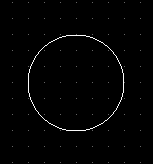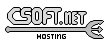Agar 1.7 Manual

M_Circle(3)

# SYNOPSIS

```#include <agar/core.h>
#include <agar/gui.h>
#include <agar/math/m.h>
```

# DESCRIPTIONThe M_Circle2 structure describes a circle in R2 in terms of an origin point p and radius r:
```typedef struct m_circle2 {
M_Vector2 p;
M_Real r;
} M_Circle2;
```

Similarly, M_Circle3 describes a circle in R3:
```typedef struct m_circle3 {
M_Vector3 p;
M_Real r;
} M_Circle3;
```

# INITIALIZATION

M_Circle2 M_CircleFromPt (M_Vector2 p, M_Real r)

void M_CircleWrite2 (AG_DataSource *ds, M_Circle2 *C)

void M_CircleWrite3 (AG_DataSource *ds, M_Circle3 *C)

M_Circle2 M_CIRCLE2_INITIALIZER (M_Real x, M_Real y, M_Real r)

M_Circle3 M_CIRCLE3_INITIALIZER (M_Real x, M_Real y, M_Real z, M_Real r)

The M_CircleFromPt() function returns a M_Circle2 describing a circle of radius r centered at point p.

The M_CircleRead() and M_CircleWrite() functions read or write a circle structure from/to an AG_DataSource(3).

The macros M_CIRCLE2_INITIALIZER() and M_CIRCLE3_INITIALIZER() expand to static initializers for M_Circle2 and M_Circle3, respectively.

# COMPUTATIONS

M_Real M_CirclePointDistance2 (M_Circle2 C, M_Vector2 p)

M_GeomSet2 M_IntersectCircleCircle2 (M_Circle2 C1, M_Circle2 C2)

M_GeomSet2 M_IntersectCircleLine2 (M_Circle2 C, M_Line2 L)

The M_CirclePointDistance2() routine computes the minimal distance between a circle C and a point p.

M_IntersectCircleCircle2() computes the intersection of two circles in R2 and returns an M_GeomSet2(3) describing the intersection. The returned set may be:
• Two points.
• One point (circles are tangent up to machine precision).
• A circle (circles are equivalent).
• The empty set.

The M_IntersectCircleCircle2() function computes the intersection of a circle and a line in R2. The returned set may be:
• Two points.
• One point (line is tangent up to machine precision).
• The empty set.www.libAgar.org is © 2022 Julien Nadeau Carriere <vedge@csoft.net>.